NEET  >  NCERT Solutions: Dual Nature of Radiation and Matter- 3

# NCERT Solutions: Dual Nature of Radiation and Matter- 3 Notes | Study Additional Documents & Tests for NEET - NEET

## Document Description: NCERT Solutions: Dual Nature of Radiation and Matter- 3 for NEET 2022 is part of Additional Documents & Tests for NEET preparation. The notes and questions for NCERT Solutions: Dual Nature of Radiation and Matter- 3 have been prepared according to the NEET exam syllabus. Information about NCERT Solutions: Dual Nature of Radiation and Matter- 3 covers topics like and NCERT Solutions: Dual Nature of Radiation and Matter- 3 Example, for NEET 2022 Exam. Find important definitions, questions, notes, meanings, examples, exercises and tests below for NCERT Solutions: Dual Nature of Radiation and Matter- 3.

Introduction of NCERT Solutions: Dual Nature of Radiation and Matter- 3 in English is available as part of our Additional Documents & Tests for NEET for NEET & NCERT Solutions: Dual Nature of Radiation and Matter- 3 in Hindi for Additional Documents & Tests for NEET course. Download more important topics related with notes, lectures and mock test series for NEET Exam by signing up for free. NEET: NCERT Solutions: Dual Nature of Radiation and Matter- 3 Notes | Study Additional Documents & Tests for NEET - NEET
 1 Crore+ students have signed up on EduRev. Have you?

Ques 11.29:
The work function for the following metals is given:

Na: 2.75 eV; K: 2.30 eV; Mo: 4.17 eV; Ni: 5.15 eV. Which of these metals will not give photoelectric emission for a radiation of wavelength 3300 Å from a He-Cd laser placed 1 m away from the photocell? What happens if the laser is brought nearer and placed 50 cm away?
Ans: Mo and Ni will not show photoelectric emission in both cases

Wavelength for a radiation, λ = 3300 Å = 3300 × 10−10 m
Speed of light, c = 3 × 108 m/s
Planck’s constant, h = 6.6 × 10−34 Js
The energy of incident radiation is given as: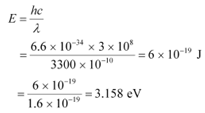It can be observed that the energy of the incident radiation is greater than the work function of Na and K only. It is less for Mo and Ni. Hence, Mo and Ni will not show photoelectric emission.

If the source of light is brought near the photocells and placed 50 cm away from them, then the intensity of radiation will increase. This does not affect the energy of the radiation. Hence, the result will be the same as before. However, the photoelectrons emitted from Na and K will increase in proportion to intensity.

Ques 11.30:
Light of intensity 10−5 W m−2 falls on a sodium photo-cell of surface area 2 cm2. Assuming that the top 5 layers of sodium absorb the incident energy, estimate time required for photoelectric emission in the wave-picture of radiation. The work function for the metal is given to be about 2 eV. What is the implication of your answer?
Ans: Intensity of incident light, I = 10−5 W m−2
Surface area of a sodium photocell, A = 2 cm2 = 2 × 10−4 m2
Incident power of the light, P = I × A
= 10−5 × 2 × 10−4
= 2 × 10−9 W
Work function of the metal,  φ= 2 eV
= 2 × 1.6 × 10−19
= 3.2 × 10−19 J
Number of layers of sodium that absorbs the incident energy, n = 5

We know that the effective atomic area of a sodium atom, Ae is 10−20 m2.

Hence, the number of conduction electrons in n layers is given as: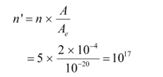The incident power is uniformly absorbed by all the electrons continuously. Hence, the amount of energy absorbed per second per electron is: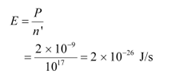Time required for photoelectric emission: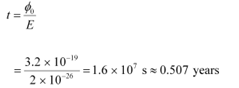The time required for the photoelectric emission is nearly half a year, which is not practical. Hence, the wave picture is in disagreement with the given experiment.

Ques 11.31:
Crystal diffraction experiments can be performed using X-rays, or electrons accelerated through appropriate voltage. Which probe has greater energy? (For quantitative comparison, take the wavelength of the probe equal to 1 Å, which is of the order of inter-atomic spacing in the lattice) (me= 9.11 × 10−31 kg).

Ans: An X-ray probe has a greater energy than an electron probe for the same wavelength.

Wavelength of light emitted from the probe, λ = 1 Å = 10−10 m

Mass of an electron, me = 9.11 × 10−31 kg

Planck’s constant, h = 6.6 × 10−34 Js

Charge on an electron, e = 1.6 × 10−19 C

The kinetic energy of the electron is given as: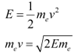Where,

v = Velocity of the electron

mev = Momentum (p) of the electron

According to the de Broglie principle, the de Broglie wavelength is given as: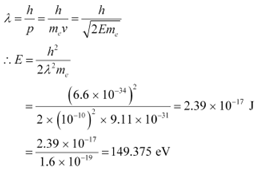Energy of a photon,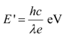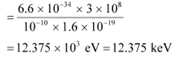Hence, a photon has a greater energy than an electron for the same wavelength.

Ques 11.32:
(a) Obtain the de Broglie wavelength of a neutron of kinetic energy 150 eV. As you have seen in Exercise 11.31, an electron beam of this energy is suitable for crystal diffraction experiments. Would a neutron beam of the same energy be equally suitable? Explain. (mn= 1.675 × 10−27 kg)

(b) Obtain the de Broglie wavelength associated with thermal neutrons at room temperature (27 ºC). Hence explain why a fast neutron beam needs to be thermalised with the environment before it can be used for neutron diffraction experiments.

Ans: (a) De Broglie wavelength =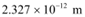; neutron is not suitable for the diffraction experiment

Kinetic energy of the neutron, K = 150 eV

= 150 × 1.6 × 10−19

= 2.4 × 10−17 J

Mass of a neutron, mn = 1.675 × 10−27 kg

The kinetic energy of the neutron is given by the relation: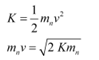Where,
v = Velocity of the neutron
mnv = Momentum of the neutron
De-Broglie wavelength of the neutron is given as: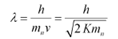It i\$ clear that wavelength is inversely proportional to the square root of mass. Hence, wavelength decreases with increase in mass and vice versa.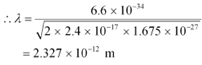It is given in the previous problem that the inter-atomic spacing of a crystal is about 1 Å, i.e., 10−10 m. Hence, the inter-atomic spacing is about a hundred times greater. Hence, a neutron beam of energy
150 eV is not suitable for diffraction experiments.

(b) De Broglie wavelength =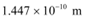Room temperature, T = 27°C = 27 + 273 = 300 K

The average kinetic energy of the neutron is given as: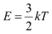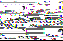Where,
k = Boltzmann constant = 1.38 × 10−23 J mol−1 K−1

The wavelength of the neutron is given as: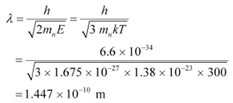This wavelength is comparable to the inter-atomic spacing of a crystal. Hence, the high-energy neutron beam should first be thermalised, before using it for diffraction.

Ques 11.33:
An electron microscope uses electrons accelerated by a voltage of 50 kV. Determine the de Broglie wavelength associated with the electrons. If other factors (such as numerical aperture, etc.) are taken to be roughly the same, how does the resolving power of an electron microscope compare with that of an optical microscope which uses yellow light?

Ans: Electrons are accelerated by a voltage, V = 50 kV = 50 × 103 V
Charge on an electron, e = 1.6 × 10−19 C
Mass of an electron, me = 9.11 × 10−31 kg
Wavelength of yellow light = 5.9 × 10−7 m
The kinetic energy of the electron is given as:

E = eV
= 1.6 × 10−19 × 50 × 103
= 8 × 10−15 J

De Broglie wavelength is given by the relation: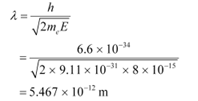This wavelength is nearly 105 times less than the wavelength of yellow light.

The resolving power of a microscope is inversely proportional to the wavelength of light used. Thus, the resolving power of an electron microscope is nearly 105 times that of an optical microscope.

Ques 11.34:
The wavelength of a probe is roughly a measure of the size of a structure that it can probe in some detail. The quark structure of protons and neutrons appears at the minute length-scale of 10−15 m or less. This structure was first probed in early 1970’s using high energy electron beams produced by a linear accelerator at Stanford, USA. Guess what might have been the order of energy of these electron beams. (Rest mass energy of electron = 0.511 MeV.)

Ans: Wavelength of a proton or a neutron, λ ≈ 10−15 m
Rest mass energy of an electron:
m0c2 = 0.511 MeV
= 0.511 × 106 × 1.6 × 10−19
= 0.8176 × 10−13 J
Planck’s constant, h = 6.6 × 10−34 Js

Speed of light, c = 3 × 108 m/s

The momentum of a proton or a neutron is given as: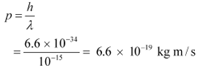The relativistic relation for energy (E) is given as: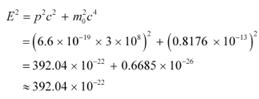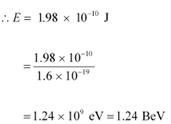Thus, the electron energy emitted from the accelerator at Stanford, USA might be of the order of 1.24 BeV.

Ques 11.35:

Find the typical de Broglie wavelength associated with a He atom in helium gas at room temperature (27 ºC) and 1 atm pressure; and compare it with the mean separation between two atoms under these conditions.

Ans: De Broglie wavelength associated with He atom =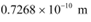Room temperature, T = 27°C = 27 273 = 300 K

Atmospheric pressure, P = 1 atm = 1.01 × 105 Pa

Atomic weight of a He atom = 4

Avogadro’s number, NA = 6.023 × 1023

Boltzmann constant, k = 1.38 × 10−23 J mol−1 K−1

Average energy of a gas at temperature T,is given as: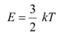De Broglie wavelength is given by the relation: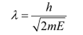Where,

m = Mass of a He atom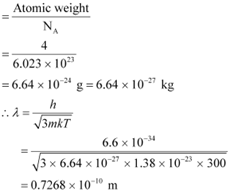We have the ideal gas formula:

PV = RT

PV = kNT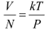Where,

V = Volume of the gas

N = Number of moles of the gas

Mean separation between two atoms of the gas is given by the relation: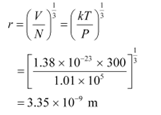Hence, the mean separation between the atoms is much greater than the de Broglie wavelength.

Ques 11.36:

Compute the typical de Broglie wavelength of an electron in a metal at 27 ºC and compare it with the mean separation between two electrons in a metal which is given to be about 2 × 10−10 m.

[Note: Exercises 11.35 and 11.36 reveal that while the wave-packets associated with gaseous molecules under ordinary conditions are non-overlapping, the electron wave-packets in a metal strongly overlap with one another. This suggests that whereas molecules in an ordinary gas can be distinguished apart, electrons in a metal cannot be distinguished apart from one another. This indistinguishibility has many fundamental implications which you will explore in more advanced Physics courses.]

Ans: Temperature, T = 27°C = 27 273 = 300 K

Mean separation between two electrons, r = 2 × 10−10 m

De Broglie wavelength of an electron is given as: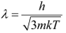Where,

h = Planck’s constant = 6.6 × 10−34 Js

m = Mass of an electron = 9.11 × 10−31 kg

k = Boltzmann constant = 1.38 × 10−23 J mol−1 K−1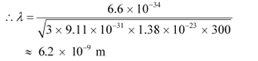Hence, the de Broglie wavelength is much greater than the given inter-electron separation.

Ques 11.37:

Answer the following questions:

(a) Quarks inside protons and neutrons are thought to carry fractional charges [( 2/3)e ; (−1/3)e]. Why do they not show up in Millikan’s oil-drop experiment?

(b) What is so special about the combination e/m? Why do we not simply talk of e and m separately?

(c) Why should gases be insulators at ordinary pressures and start conducting at very low pressures?

(d) Every metal has a definite work function. Why do all photoelectrons not come out with the same energy if incident radiation is monochromatic? Why is there an energy distribution of photoelectrons?

(e) The energy and momentum of an electron are related to the frequency and wavelength of the associated matter wave by the relations:

E = hν, p =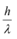But while the value of λ is physically significant, the value of ν (and therefore, the value of the phase speed νλ) has no physical significance. Why?

Ans: (a) Quarks inside protons and neutrons carry fractional charges. This is because nuclear force increases extremely if they are pulled apart. Therefore, fractional charges may exist in nature; observable charges are still the integral multiple of an electrical charge.

(b) The basic relations for electric field and magnetic field are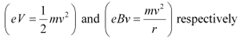These relations include e (electric charge), v (velocity), m (mass), V (potential), r (radius), and B (magnetic field). These relations give the value of velocity of an electron as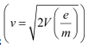and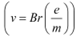It can be observed from these relations that the dynamics of an electron is determined not by e and m separately, but by the ratio e/m.

(c) At atmospheric pressure, the ions of gases have no chance of reaching their respective electrons because of collision and recombination with other gas molecules. Hence, gases are insulators at atmospheric pressure. At low pressures, ions have a chance of reaching their respective electrodes and constitute a current. Hence, they conduct electricity at these pressures.

(d) The work function of a metal is the minimum energy required for a conduction electron to get out of the metal surface. All the electrons in an atom do not have the same energy level. When a ray having some photon energy is incident on a metal surface, the electrons come out from different levels with different energies. Hence, these emitted electrons show different energy distributions.

(e) The absolute value of energy of a particle is arbitrary within the additive constant. Hence, wavelength (λ) is significant, but the frequency (ν) associated with an electron has no direct physical significance.

Therefore, the product νλ(phase speed)has no physical significance.

Group speed is given as: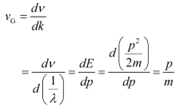This quantity has a physical meaning.

The document NCERT Solutions: Dual Nature of Radiation and Matter- 3 Notes | Study Additional Documents & Tests for NEET - NEET is a part of the NEET Course Additional Documents & Tests for NEET.
All you need of NEET at this link: NEET

## Additional Documents & Tests for NEET

10 videos|153 docs|55 tests
 Use Code STAYHOME200 and get INR 200 additional OFF

## Additional Documents & Tests for NEET

10 videos|153 docs|55 tests

### How to Prepare for NEET

Read our guide to prepare for NEET which is created by Toppers & the best Teachers

Track your progress, build streaks, highlight & save important lessons and more!

,

,

,

,

,

,

,

,

,

,

,

,

,

,

,

,

,

,

,

,

,

;